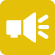## 1-Minute Survey

What Type of Investor Are You? – Take This 1-Min Survey to Find Out

Financial Literacy

# What is Sum-of-Years Digits?

There are several ways a business can depreciate an asset—namely through straight line or accelerated modes. For an accelerated depreciation schedule, sum-of-years digits is typically the most common. Sum-of-years digits is an accelerated depreciation method that applies a percentage of depreciation based on the number of years left in the asset’s useful life.

Companies often use this depreciation method to reduce the burden of bringing on a new asset in the current period and those immediately following. It’s an accounting tactic that unburdens the balance sheet, without discrediting the useful life of the asset in question. Businesses use this and other accelerated forms of depreciation to prevent balance sheet congestion. It can offer immediate relief, but has long-term implications that companies and investors need to be aware of.## What is an Accelerated Depreciation Rate?

As an accelerated depreciation rate, sum-of-years digits allows companies to write down more of an asset’s value quicker, tapering the total amount of depreciation over time. While the asset will still end up fully depreciated at the end of the schedule, this method allows a business to alleviate more of that asset from its balance sheet earlier. It’s a common accounting tactic for companies that make significant asset investments that they’ll rely on for a long period of time.

The drawback of an accelerated depreciation rate like sum-of-years digits? Writing down more of the asset’s value in earlier years can inflate the role of asset maintenance and upkeep later in the asset’s life, when the depreciation amount is lower. With a straight-line depreciation method, a standard depreciation rate can help offset higher cost of ownership in later years.

## Calculating Sum-of-Years Digits

To calculate asset depreciation using this method, you first need to estimate how many serviceable years of life the business will use the asset for. Then, you incrementally add those years together to get the depreciation rate. For example, if the business expects an asset to last six years, it would use the following calculation to get the annual depreciation rate:

1+2+3+4+5+6 = 21

In this example, 21 is the depreciation rate. To apply it, the company would divide the years remaining by the depreciation rate to get the total depreciable amount for that given year (rounding appropriately):

• Year 1. 6/21 = 29%
• Year 2. 5/21 = 24%
• Year 3. 4/21 = 19%
• Year 4. 3/21 = 14%
• Year 5. 2/21 = 9%
• Year 6. 1/21 = 5%

Though the amount differs each year, the total depreciation should equal 100% by the end of the depreciation schedule. Hence the name: sum-of-years digits.

## Strategies Behind Sum-of-Years Digits Depreciation

The sum of years expected varies greatly from asset to asset. While the strategy for accelerated depreciation remains the same, some assets will feature a longer or shorter schedule with differing degrees of tapering depreciation. For example, an asset with a six-year lifespan will see a 5% depreciation in its final year. An asset with a four-year depreciation schedule will see a 10% depreciation in its final year. Companies can use this to their advantage regarding how they account for certain assets.

• Take something like a car, for example. It’s well-known that vehicles are fast-depreciating assets that lose significant value almost immediately. In this case, an accelerated depreciation makes sense, since it paces the actual depreciation of the asset.
• Now, consider something with a very long serviceable life, like a piece of construction equipment. The company will likely carry this asset for a decade, which can hamper its balance sheet if depreciated at a constant rate. Sum-of-years digits allows the company to quickly depreciate that burden, while still gaining value from a critical asset.
• Finally, companies need to consider their financial accounting—specifically, the complete balance sheet picture. Sum-of-years can bring stability to the balance sheet year-over-year by cycling depreciation rates based on when the company acquired the asset vs. how many years it has left.

There’s a lot of opportunity in financial control and fiscal posturing when it comes to sum-of-years digits as a mode of asset depreciation, Companies need to identify the strategy that works best for the asset within the context of the balance sheet.

## Sum-of-Years Digits vs. Other Types of Depreciation

Sum-of-years digits is one of four modes of asset depreciation a business can use. The others include:

Each of these methods are allowable under Generally Accepted Accounting Principles (GAAP). The best mode of depreciation depends on the company’s financial reporting goals and the nature of the asset itself. While sum-of-years digits are appropriate for assets that depreciate rapidly, straight-line depreciation may be more applicable for assets that have better salvage value. A strategy like units of production is best-reserved for depreciating inventory. Moreover, declining balance is the most aggressive form of depreciation—even more so than sum-of-years digits.

## Making the Most of Asset Depreciation Through the Years

Every asset a company acquires is subject to depreciation. How quickly it records that depreciation is up to the business—and often, accelerating the write-down is a favorable course of action.